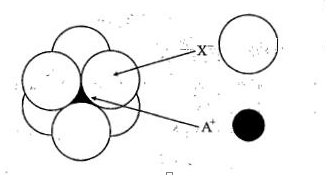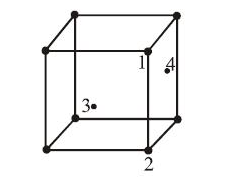If NaCl is doped with 10-4 mol % of SrCl2, the concentration of cation vacancies will be (NA = 6.023 x 1023 mol-1)

(1) 6.023 x 1015 mol-1

(2) 6.023 x 1016 mol-1

(3) 6.023 x 1017 mol-1

(4) 6.023 x 1014 mol-1

Concept Questions :-

Density/Formula/packing fraction/ Semiconductors
NEET 2021 - Achiever Batch - Aryan Raj Singh

Difficulty Level:

CsCl crystallises in body-centred cubic lattice. If 'a' is its edge length then which of the following expressions is correct?

(1) rCs+ + rCl- = 3a

(2)  rCs+ + rCl- = 3a/2

(3)  rCs+ + rCl- = ($\sqrt{3}$/2)*a

(4) rCs+ + rCl-$\sqrt{3a}$

Concept Questions :-

Introduction and Type of Crystal System
NEET 2021 - Achiever Batch - Aryan Raj Singh

Difficulty Level:

The phenomenon in which polar crystals on heating produce electricity is called:-
1. pyro-electricity
2. piezo-electricity
3. ferro-electricity
4. ferri-electricity

Concept Questions :-

Magnetic Behaviour of an Element/Compound
NEET 2021 - Achiever Batch - Aryan Raj Singh

Difficulty Level:

Metals have conductivity of the order of (ohm-1 cm-1):-

(1)1012

(2)108

(3)102

(4)10-6

Concept Questions :-

Density/Formula/packing fraction/ Semiconductors
NEET 2021 - Achiever Batch - Aryan Raj Singh

Difficulty Level:

Total volume of atoms present in a face centred cubic unit cell of a metal is ( r is atomic radius):

(1) 20/3 $\pi$r3

(2)  24/3 $\pi$r3

(3)  12/3 $\pi$r3

(4)  16/3 $\pi$r3

Concept Questions :-

NEET 2021 - Achiever Batch - Aryan Raj Singh

Difficulty Level:

Lithium borohydride (LiBH4), crystallises in an orthorhombic system with 4 molecules per unit cell. The unit cell dimensions are:- a = 6.81 Å , b = 4.43 Å ,c = 7.17 Å. If the molar mass of LiBH4 is
21.76 g mol-1.The density of the crystal is:-

1. 0.668 gcm-3
2. 0.585 gcm2
3. 1.23 gcm-3

4. None of these

Concept Questions :-

Density/Formula/packing fraction/ Semiconductors
NEET 2021 - Achiever Batch - Aryan Raj Singh

Difficulty Level:

The arrangement of Xions around A+ ion in solid AX is given in the figure (not drawn to scale).
If the radius of X is 250 pm,the radius of A+ is:(1)104 pm

(2)125 pm

(3)183 pm

(4) 57 pm

Concept Questions :-

NEET 2021 - Achiever Batch - Aryan Raj Singh

Difficulty Level:

In an f.c.c. unit cell. atoms are numbered as shown below.The atoms not touching each other are (Atom numbered 3 is face centre of front face).(1) 3&4

(2) 1&3

(3) 1&2

(4) 2&4

Concept Questions :-

Introduction and Type of Crystal System
NEET 2021 - Achiever Batch - Aryan Raj Singh

Difficulty Level:

A solid has a bcc structure. If the distance of closest approach between the two atoms is 1.73Å . The edge length of the cell is:

(1) 200pm

(2) $\sqrt{3}}{\sqrt{2}}$ pm

(3) 142.2 pm

(4) $\sqrt{2}$pm

Concept Questions :-

Density/Formula/packing fraction/ Semiconductors
NEET 2021 - Achiever Batch - Aryan Raj Singh

Difficulty Level:

Which is covalent solid?

(1) Fe2O3

(2) Diamond

(3) Graphite

(4) All of these

Concept Questions :-

Introduction and Type of Crystal System